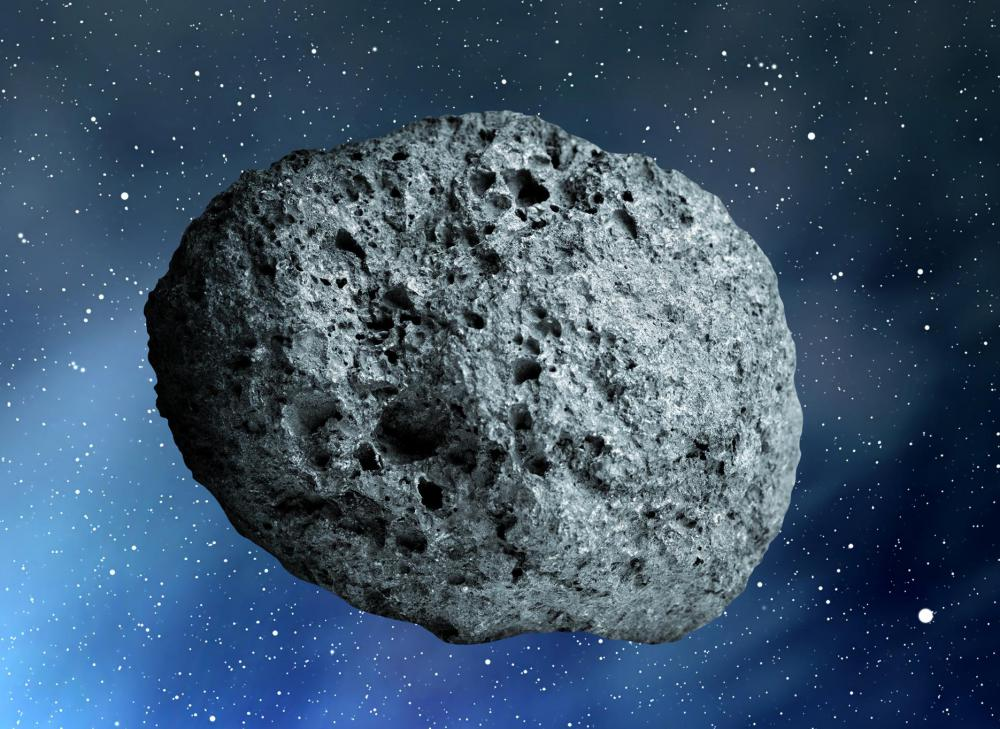# What is Kepler's Third Law?

J.A. Moles
J.A. MolesAs asteroids and other objects come closer to the sun, they speed up.

Kepler's third law of planetary motion states that the square of each planet's orbital period, represented as P2, is proportional to the cube of each planet’s semi-major axis, R3. A planet's orbital period is simply the amount of time in years it takes for one complete revolution. A semi-major axis is a property of all ellipses and is the distance from the center of the ellipse to point on the orbit that is furthest away from the center.

Astronomer and mathematician Johannes Kepler (1571-1630) developed his three laws of planetary motion with respect to any two objects in orbit, and it makes no difference if those two objects are stars, planets, comets or asteroids. This is mostly true for any two relatively massive objects in space. Kepler's laws changed the way humans studied the movements of celestial bodies.

The following example can be used to demonstrate the properties of each ratio with respect to Kepler's third law. If P1 represents Planet A's orbital period and R1 represents Planet A's semi-major axis; P2 represents Planet B's orbital period and R2 represents Planet B's semi-major axis; then the ratio of (P1)2/(P2)2, that is, the square of each planet's orbital period, equals the ratio of (R1)3/(R2)3, the cube of each planet's semi-major axis. Thus, as an expression, Kepler's third law shows that (P1)2/(P2)2 = (R1)3/(R2)3.

Instead of ratios or proportions, Kepler's third law can be summed up using time and distance. As planets, comets, or asteroids get closer to the Sun, their speeds increase; when planets, comets, or asteroids get farther away, their speeds decrease. Therefore, one body's speed increase is similar to another body's speed increase when both of their distances — their semi-major axes — are taken into consideration. This is why Mercury, the inner-most planet, revolves so quickly and Pluto, formerly considered the outer-most planet, revolves so slowly.

In a real world example using Mercury and Pluto, note the larger numbers are that of Pluto and remember (P1)2/(P2)2 = (R1)3/(R2)3. In this case, (0.240)2/(249)2 = (0.39)3/(40)3. Therefore, 9.29 x 10-7 = 9.26 x 10-7.

Mercury is always near the Sun, so its velocity is high. Pluto is always away from the Sun, so its velocity is slow, but neither object's velocity is constant. Even though Mercury is close by and Pluto is far away, both have times during their orbital periods of increasing and decreasing velocity. Regardless of differences, the square of each planet's orbital period is proportional to the cube of each planet's semi-major axis.

## You might also Like## Discuss this Article

Forgot password?
###### Register:
•As asteroids and other objects come closer to the sun, they speed up.